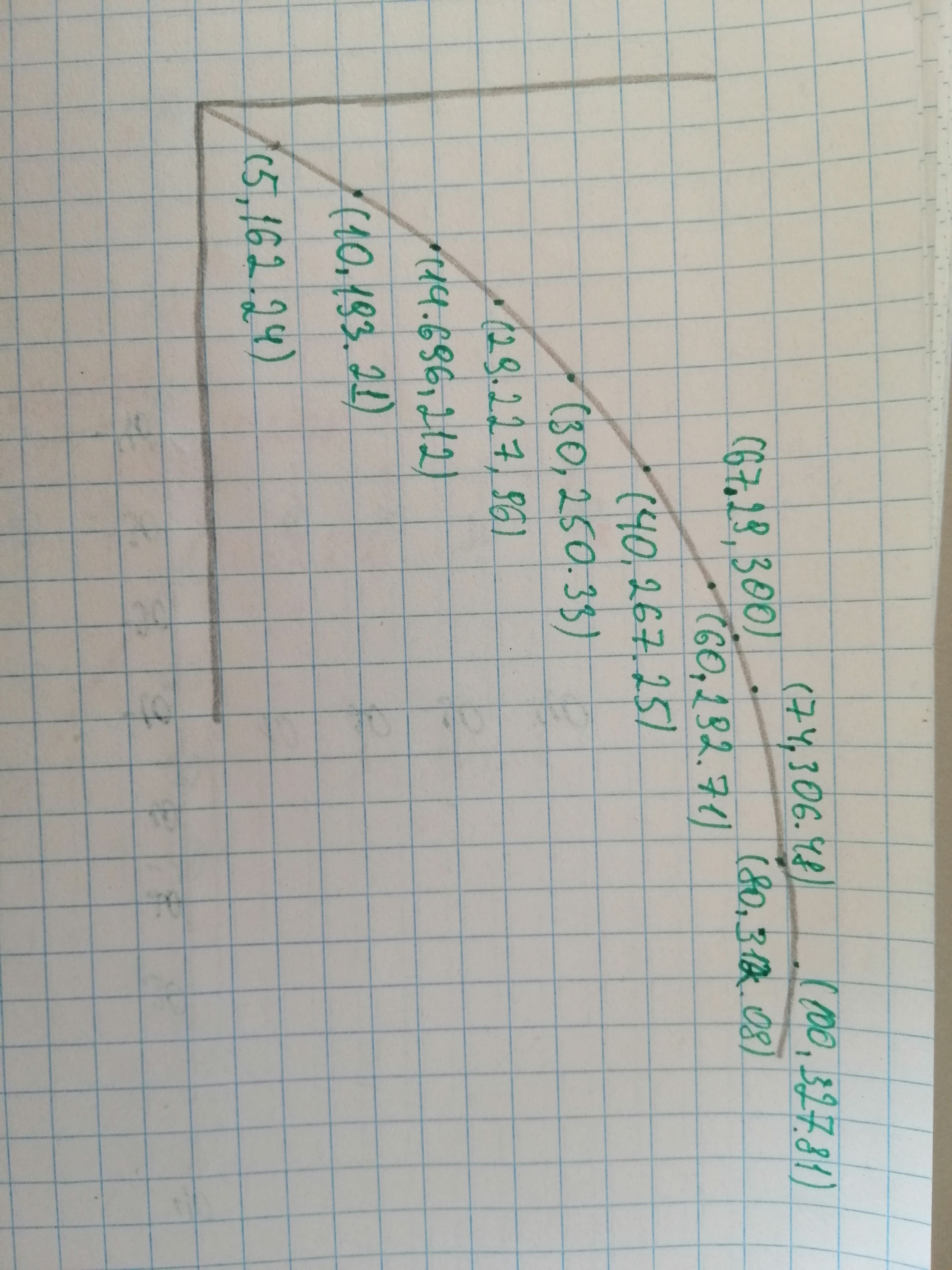# The table shows the temperatures T (in degrees Fahrenheit) at which water boils at selected pressures p (in pounds per square inch).Nannie Mack 2021-01-25 Answered

The table shows the temperatures T (in degrees Fahrenheit) at which water boils at selected pressures p (in pounds per square inch). A model that approximates the datais: a) Use a graphing untility to plot the data and graph the model in the same veiwing window. How well does the model fit the data? b) Use the graph to estimate the pressure at which the boiling point of water is  ${300}^{\circ }$ F. c) Calculate T when the pressure is 74 pounds per square inch. Verify your answer graphically.

You can still ask an expert for help

• Questions are typically answered in as fast as 30 minutes

Solve your problem for the price of one coffee

• Math expert for every subject
• Pay only if we can solve itopsadnojD

a) To approximate the data and the table provided in the question shown below.

Graph: Sketch the graph using graphing utility.

Step 1: Press WINDOW button to access the Window editor.

Step 2: Press Y= button.

Step 3: Enter the data which is required to graph. Step 4: Press GRAPH button to graph the function. The graph is obtained as:Interpretation: From the graph it is observed that graph shows the curve.

b) Calculate: From the above graph the pressure required is 67.29 pounds per square inch.

c) Calculation:

$T\left(74\right)=306.4845$